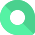# ACV Growth Rate

## What is ACV Growth Rate?

ACV Growth Rate is the change in the average contract value over a given time period when compared to the previous time period, typically represented by a percentage.

Alternate names: Annual Contract Value Growth Rate

### How to calculate ACV Growth Rate

ƒ (Sum(ACV end of period) – Sum(ACV beginning of period))/Sum(ACV beginning of period)
ƒ (Sum(ACV end of period)/Sum(ACV beginning of period) - 1)

### Example

A SaaS company had an ACV of \$40,000 at the beginning of last year. By strategically upselling, the company brought the ACV up to \$60,000 at the end of the year. This means that the company’s ACV Growth Rate in this scenario is (\$60,000 - \$40,000) / \$40,000 which is 50%.